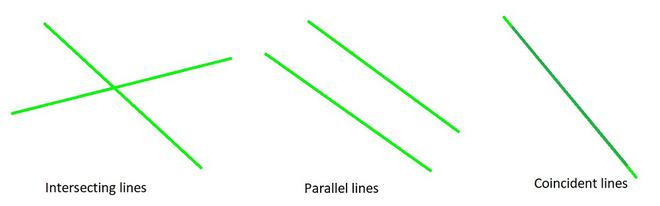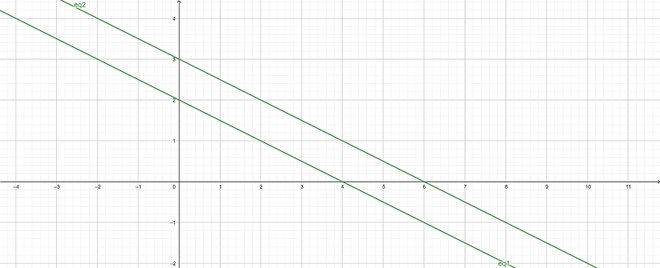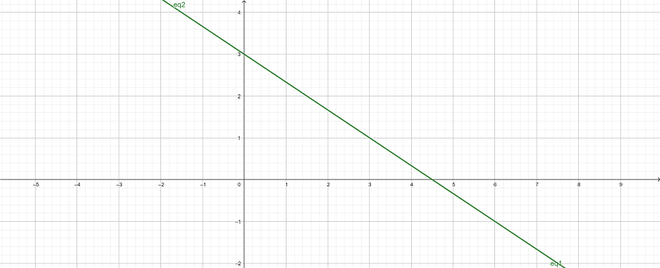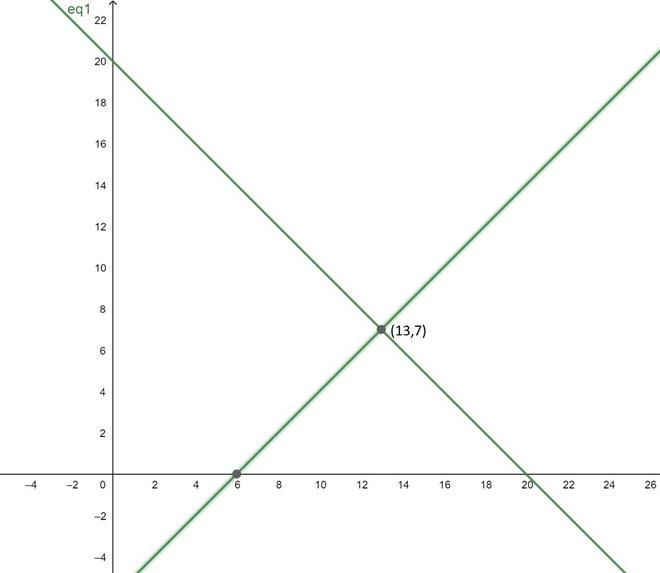GeeksforGeeks App
Open AppBrowser
Continue

# Number of Solutions to a System of Equations Algebraically

A statement that two mathematical expressions of one or more variables are identical is called an equation. Linear equations are those in which the powers of all the variables concerned are equal. A linear equation’s degree is always one. A solution of the simultaneous pair of linear equations is a pair of values of variables “x” and “y” that satisfy all equations in the specified set of equations.

### Pair of Linear Equations in Two Variables

An equation that can be put in the form ax + by + c = 0, where a, b and c are real numbers, and a and b are not both zero, is called a linear equation in two variables x and y. (or this condition should be satisfied where a and b are not both zero by a2+ b2 ≠ 0).

e.g.: Consider a pair of linear equations in two variables be 3x + 2y = 6,

Substitute x = 2 and y = 0 in the left-hand side (LHS) as:

⇒ LHS = 3(2) + 2(0)

= 6 + 0

= 6 = RHS

Therefore, x = 2 and y = 0 is a solution of the equation 2x + 3y = 6.

Now, if x = 1 and y = 1 is substituted in the equation 2x + 3y = 6, then:

LHS = 3(1) + 2(1)

= 3 + 2

= 5 ≠ RHS

Therefore, x = 1 and y = 1 is not a solution of the equation.

Algebraically, this indicates that the point (2, 0) lies on the line representing the equation 3x + 2y = 6, and the point (1, 1) does not lie on it.

Thus, every solution that satisfies the equation is a point on the line representing it.

Two linear equations like this, having two variables x and y. Equations like these are called a pair of linear equations in two variables

Algebraically, the general form for a pair of linear equations in two variables x and y is:

a1 x + b1 y + c1 = 0 and

a2 x + b2 y + c2 = 0

where a1, b1, c1, a2, b2, c2 are all real numbers (∈ R) and a12 + b12 ≠ 0, a22+ b2 2 ≠ 0.

### Different cases possible for the pair of linear equations in two variables

There are three different cases for three different types of lines: intersecting, parallel and coincident lines to determine the pair of linear equations in two variables.• The lines may intersect each other at a single point.

As a result, the pair of equations has a unique solution (consistent pair of equations).

e.g.: 2x – 4y = 0 and 6x + 8y – 40 = 0Intersecting lines: 2x – 4y = 0 and 6x + 8y – 40 = 0

• The lines may be parallel to each other.

As a result, the equations have no solution (inconsistent pair of equations).

e.g.: 2x + 4y – 8 = 0 and 4x + 8y – 24 = 0Parallel lines: 2x + 4y – 8 = 0 and 4x + 8y – 24 = 0

• The lines may be coincident

As a result, the equations have infinitely many solutions (dependent or consistent pair of equations)

e.g.: 4x + 6y – 18 = 0 and 8x + 12y – 36 = 0Intersecting lines: 4x + 6y – 18 = 0 and 8x + 12y – 36 = 0

Now, Lets assume the pair of equations: a1x + b1y + c1 = 0 and a2x + b2y + c2 = 0 now their graphical and algebraic interpretation corresponding to the comparing ratios is given as:

### Sample Problems

Problem 1: Find out the graphical representation and specify the number of solutions of the following pairs of linear equations: 8x – 4y + 10 = 0 and 4x – 2y + 9 = 0.

Solution:

For the given pair of linear equations:

a1 = 8, b1 = -4, c1 = 10 and

a2 = 4, b2 = -2, c2 = 9

Therefore,

a1 / a2 = 8 / 4 = 2

b1 / b2 = -4 / -2 = 2 and

c1 / c2 = 10 / 9

This implies that:Hence, the given pairs of linear equations have no solution and the lines are parallel and never intersect each other.

Problem 2: Determine the number of solutions of the following pairs of linear equations: 6x + 2y = 4 and 7x – 3y = 13.

For the given pair of linear equations:

a1 = 6, b1 = 2, c1 = -4 and

a2 = 7, b2 = -3, c2 = -13

Therefore,

a1 / a2 = 6 / 7

b1 / b2 = 2 / -3 and

c1 / c2 = -4 / -13

This implies that:Hence, the given pairs of linear equations have a unique solution and the lines intersect each other at exactly one point.

Problem 3: Determine the graphical representation and the number of solutions of the following pairs of linear equations: 6x – 5y = 11; – 12x +10y = –22.

For the given pair of linear equations:

a1 = 6, b1 = -5, c1 = -11 and

a2 = -12, b2 = 10, c2 = 22

Therefore,

a1 / a2 = 6 / -12 = -1 / 2

b1 / b2 = -5 / 10 = -1 / 2 and

c1 / c2 = -11 / 22

This implies that:Hence, the given pairs of linear equations have infinite many solutions and the lines are coincident.

Problem 4: Make the pair of linear equations in the following word problems, and find their solutions graphically.

There are 20 students in a class. If the number of boys is 6 more than the number of girls, find the number of boys and girls in the class.

Solution:

Consider, the number of girls be x and the number of boys be y.

Therefore, according to the given conditions:

x + y = 20                                                                                                                     ……(1)

x – y = 6                                                                                                                       ……(2)

In order, to construct the graph the solutions of the given equation is needed to be determined.

For equation (1): x + y = 20, the solutions are:

For equation (2): x – y = 6, So, the solutions are:

Thus, plotting the above points for equation (1) and (2) as:Now, from the graph it can be concluded that the given lines intersect each other at point (13, 7).

Hence, the number of girls are 7 and number of boys are 13 in a class.

Problem 5: Determine other linear equation in two variables such that it should form:

(i) intersecting lines with the line 6x + 7y – 8 = 0.

(ii) parallel lines with the line 4x-5y-8=0.

Solution:

(i)

In order to make a pair of lines intersect, they must satisfy the given conditions:On rearranging the above expression as:Therefore, in the equation the ratio should not be 6/7.

So, another equation can be 5x – 9y + 9 = 0

where the ratio is 5 / -9 and(ii)

In order to make a pair of lines parallel, they should satisfy the given conditions:On rearranging the above expression as:Therefore, the required equation a2 / b2 should be in ratio of 4 / -5 and b2 / c2 should not be equal to -5 / -8.

So, another equation can be 8x – 10y + 9 = 0

where the ratio a2 / b2 is 8/-10 = -8/10 andMy Personal Notes arrow_drop_up
Related Tutorials